# Valuation of shares Need for valuation 1 At

• Slides: 10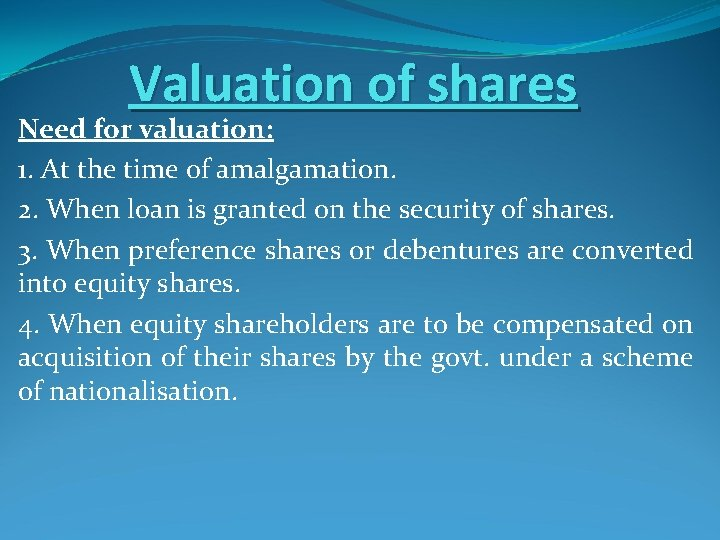Valuation of shares Need for valuation: 1. At the time of amalgamation. 2. When loan is granted on the security of shares. 3. When preference shares or debentures are converted into equity shares. 4. When equity shareholders are to be compensated on acquisition of their shares by the govt. under a scheme of nationalisation.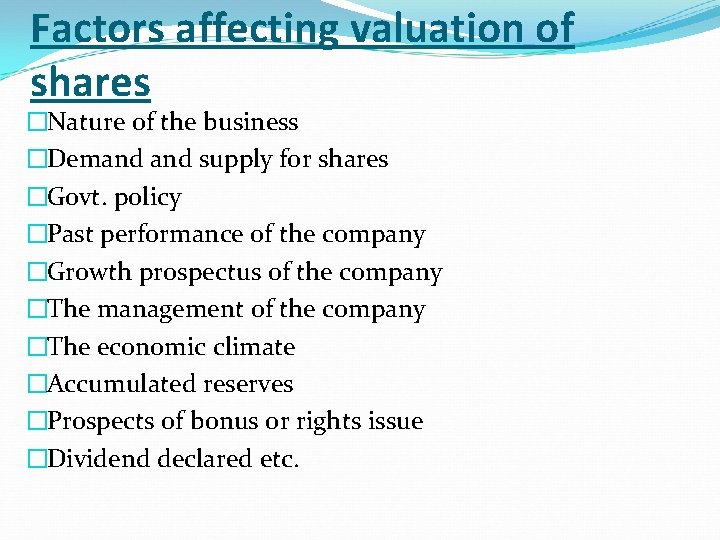Factors affecting valuation of shares �Nature of the business �Demand supply for shares �Govt. policy �Past performance of the company �Growth prospectus of the company �The management of the company �The economic climate �Accumulated reserves �Prospects of bonus or rights issue �Dividend declared etc.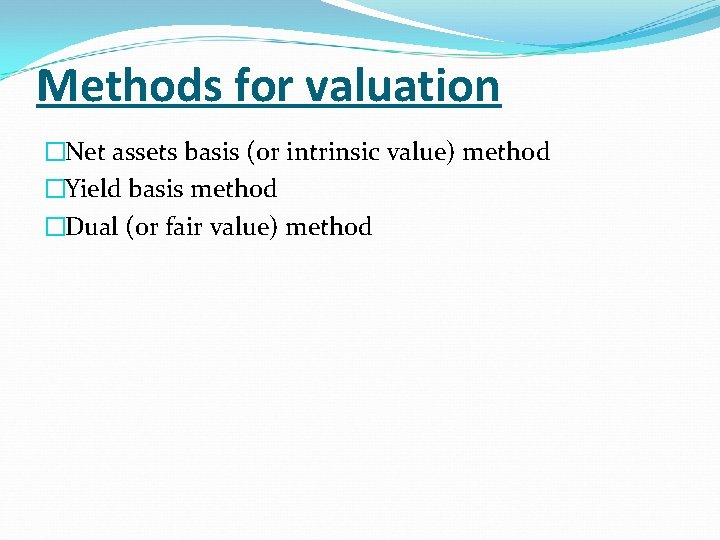Methods for valuation �Net assets basis (or intrinsic value) method �Yield basis method �Dual (or fair value) method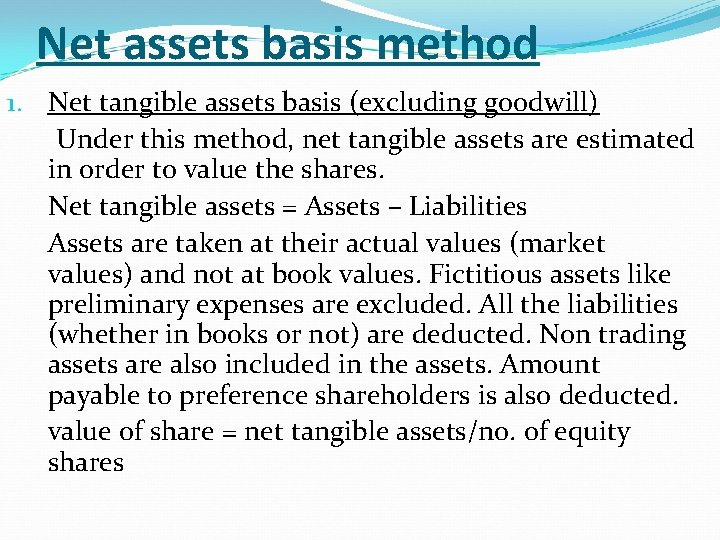Net assets basis method 1. Net tangible assets basis (excluding goodwill) Under this method, net tangible assets are estimated in order to value the shares. Net tangible assets = Assets – Liabilities Assets are taken at their actual values (market values) and not at book values. Fictitious assets like preliminary expenses are excluded. All the liabilities (whether in books or not) are deducted. Non trading assets are also included in the assets. Amount payable to preference shareholders is also deducted. value of share = net tangible assets/no. of equity shares2. Net assets (including goodwill) In this method, goodwill is included with other tangible assets for the valuation of shares. Goodwill is taken at its actual value which maybe equal to, more than or less than the book value. There maybe some value of goodwill even if it is not shown in the books. Example: Assests = Rs. 10, 000 Liabilities= Rs. 2, 000 No. of shares= 50, 000 Value of share= (10, 000 -2, 000)/50, 000= Rs. 16Yield method Following steps are taken for calculating the value of shares under this method: 1. Calculation of average expected future profits (profit available for equity shareholders). 2. Calculation of expected return. Expected return= expected profits/equity share capital *100 3. Value of share= Expected rate/normal rate *paid up value of one shareExample: 2000, 9% preference shares of Rs. 100 each: Rs. 2, 000 50, 000 equity shares of Rs. 10 each, Rs. 8 per share paid up: Rs. 4, 000 Expected profit per year before tax: Rs. 2, 18, 000 Rate of tax: 40% Transfer to general reserve every year: 20% of profit Normal rate of earning: 15%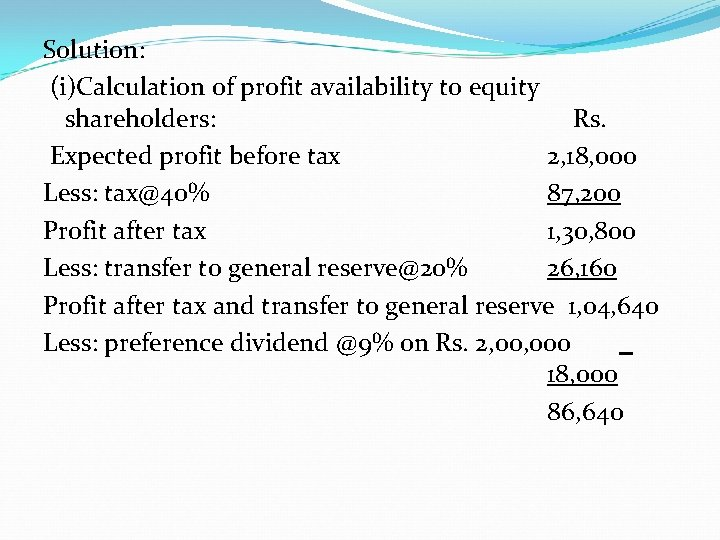Solution: (i)Calculation of profit availability to equity shareholders: Rs. Expected profit before tax 2, 18, 000 Less: [email protected]% 87, 200 Profit after tax 1, 30, 800 Less: transfer to general [email protected]% 26, 160 Profit after tax and transfer to general reserve 1, 04, 640 Less: preference dividend @9% on Rs. 2, 000 18, 000 86, 640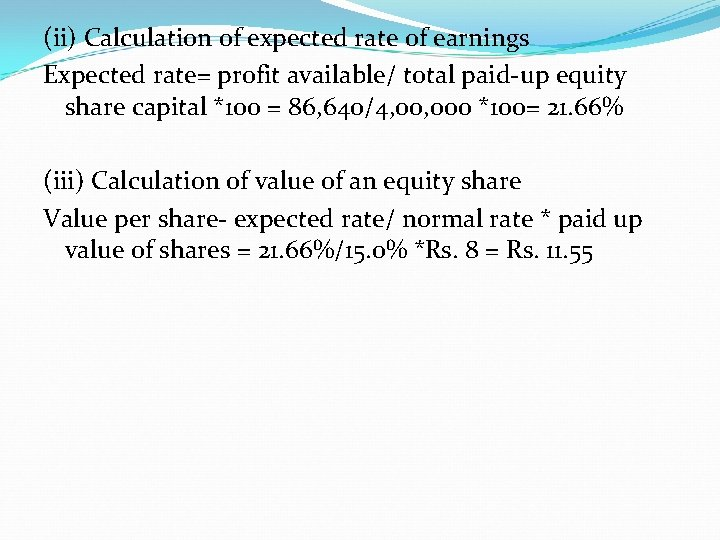(ii) Calculation of expected rate of earnings Expected rate= profit available/ total paid-up equity share capital *100 = 86, 640/4, 000 *100= 21. 66% (iii) Calculation of value of an equity share Value per share- expected rate/ normal rate * paid up value of shares = 21. 66%/15. 0% *Rs. 8 = Rs. 11. 55Dual or fair value method It is simply a combination of the previous two methods. According to this method, Value of share= net asset method value + yield method value/2 For eg: Net asset method value: Rs. 10 Yield method value: Rs. 12 Fair value: Rs. 10+Rs. 12/2 =Rs. 11